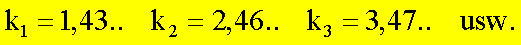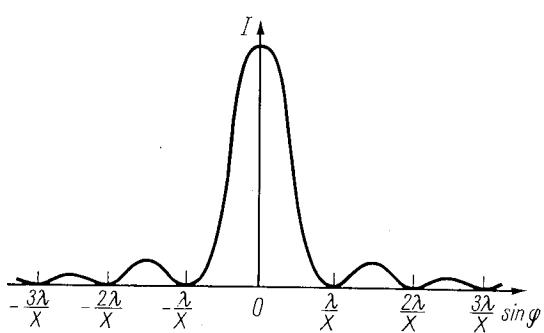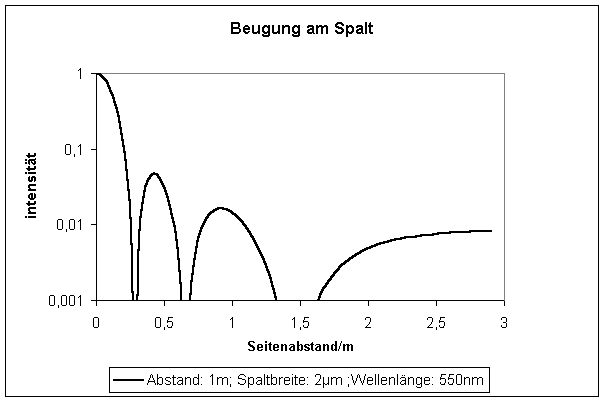# What is Fraunhofer Diffraction

Fraunhofer diffraction at the slit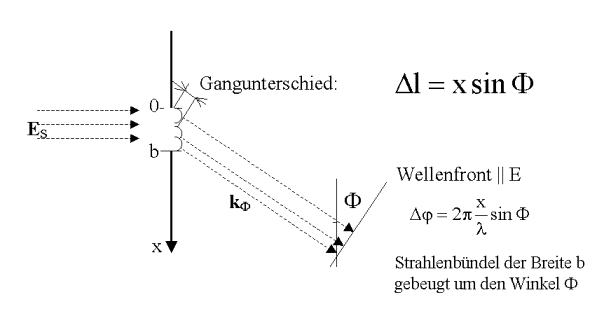Phase difference: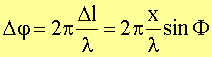Every point x of the opening is the starting point of an elementary wave (Huygens principle). The superposition of all partial beams diffracted at the angle F corresponds to a summation of the field strengths from x = 0 to x = b (with a fixed F) in the direction kF. outgoing partial waves and results in the field strength at the wave front taking into account the phase relationships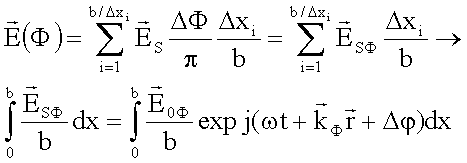The angle element DF / p corresponds to the proportion of that emanating from the gap b Elementary waveswhich is scattered in the direction of F, F + DF. The ratio (Dx / b) corresponds to a portion of the beam of width Dx passing through the gap b.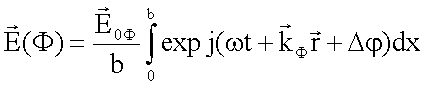Using the expression for the position-dependent phase difference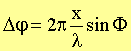we get the field strength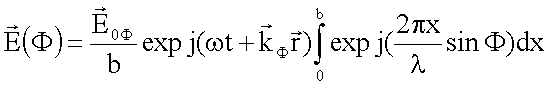This gives the amplitude of the field strength as a function of the angle F: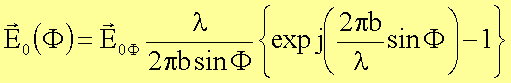The intensity is proportional to the square of the amount of the field strength: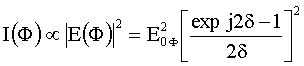By means of relationships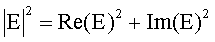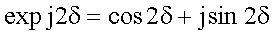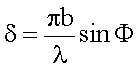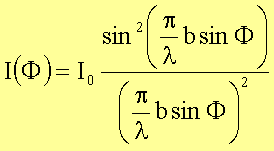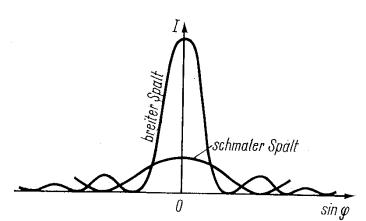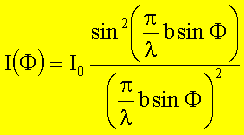### Calculation of the intensity minima

With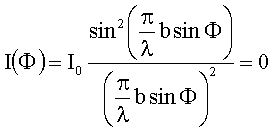follows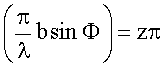The condition for intensity minima results from this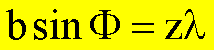(Note: For diffraction at the grating, this is the condition for intensity maxima!)

### It's the problem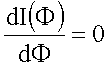to solve for the occurrence of relative maxima.

The intensity equation can be in the simplified form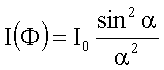write down. By differentiating for a and setting to zero it follows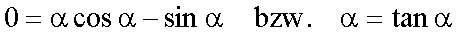So the transcendent equation must be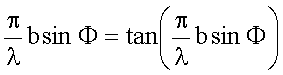be solved.

This equation has the solutions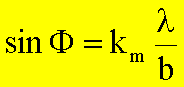Where the coefficients km Solutions to the equation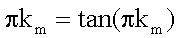are with